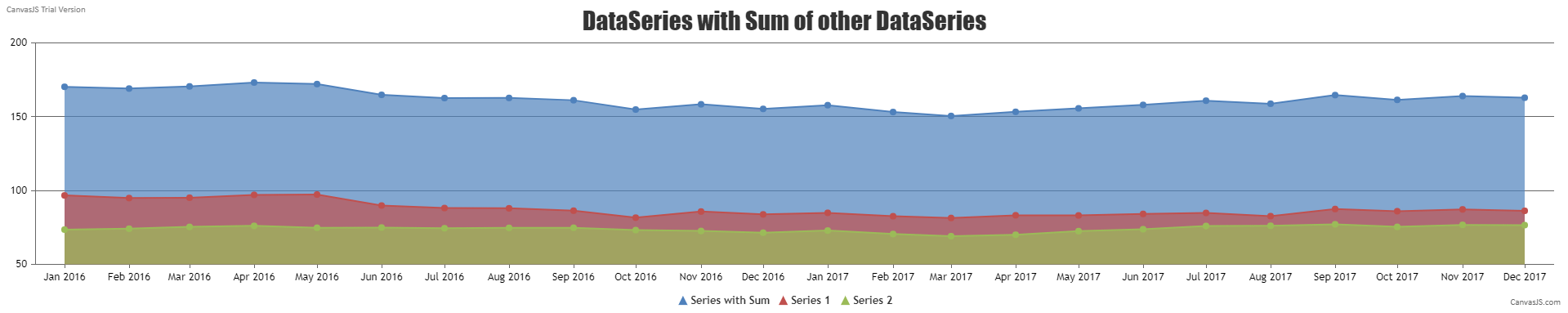Home Forums Chart Support Multi Series Area Charts

# Multi Series Area Charts

Viewing 3 posts - 1 through 3 (of 3 total)
• #32895

Does this area chart –

https://canvasjs.com/php-charts/multi-series-area-chart/

support the sum of the two y axis data points as a 3rd data line ?

#32897

Would it be as simple as adding (3) –

\$dataPoints3 = array( …

????

#32927

It is possible to create a new dataPoints array by summing up each y value of the first 2 dataPoints array as shown below –

``````\$dataPoints3 = array();
\$sumDataPoints = array();

foreach(\$dataPoints1 as \$dps) {
\$sumDataPoints[\$dps["x"]] = array_key_exists(\$dps["x"], \$sumDataPoints) ? \$sumDataPoints[\$dps["x"]] + \$dps["y"] : \$dps["y"];
}
foreach(\$dataPoints2 as \$dps) {
\$sumDataPoints[\$dps["x"]] = array_key_exists(\$dps["x"], \$sumDataPoints) ? \$sumDataPoints[\$dps["x"]] + \$dps["y"] : \$dps["y"];
}

foreach(\$sumDataPoints as \$x => \$dps) {
array_push(\$dataPoints3,array("x" => \$x, "y" => \$sumDataPoints[\$x]));
}``````

You can create a multi-series area chart by providing each of these dataPoints array as separate dataSeries.

Kindly take a look at this Sample php project for an example on creating a multi-series area chart with the third dataSeries being the sum of the first two dataSeries.___________